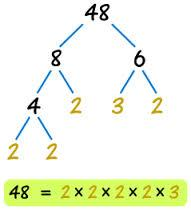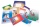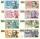# Decomposition

Make decomposition using prime numbers of number 155. Result write as prime factors (all, even multiple)

p1 =  5
p2 =  31

### Step-by-step explanation:

$155=5\cdot 31$Did you find an error or inaccuracy? Feel free to write us. Thank you!## Related math problems and questions:

• Find unknown numberWhat is the number between 50 and 55 that is divisible by 2,3,6,9?
• Prime factorsWrite 98 as a product of prime factors
• On Children'sOn Children's Day, the organizers bought 252 chewing gums, 396 candies and 108 lollipops. They want to make as many of the same packages as possible. Advise them what to put in each package and how many packages they can make this way.
• RemaindersIt is given a set of numbers { 170; 244; 299; 333; 351; 391; 423; 644 }. Divide this numbers by number 66 and determine set of remainders. As result write sum of this remainders.
• Unknown numberSamuel wrote unknown number. Then he had add 200000 to the number and the result multiply by three. When it calculated he was surprised, because the result would have received anyway, if write digit to the end of original number. Find unknown number.
• Amazing numberAn amazing number is name for such even number, the decomposition product of prime numbers has exactly three not necessarily different factors and the sum of all its divisors is equal to twice that number. Find all amazing numbers.
• School yearAt the beginning of the school year, 396 notebooks and 252 textbooks are ready to be distributed in the classroom. All pupils receive the same number of notebooks and the same amount of textbooks. How many pupils are there in the class if you know that th
• Cash backAfter a trip 24 students have left more than 650 but less than 690 CZK (Kc). How much each student receives return when the amount is divisible by the number of pupils?
• LCMWhat is the least common multiple of 5, 50, 14?
• Prime factorsFactor the number 6600 into the product of prime numbers.
• School booksAt the beginning of the school year, the teacher distributed 480 workbooks and 220 textbooks. How many pupils could have the most in the classroom?
• Prime divisorsFind 2/3 of the sum's ratio and the product of all prime divisors of the number 120.
• Reminder and quotientThere are given numbers A = 135, B = 315. Find the smallest natural number R greater than 1 so that the proportions R:A, R:B are with the remainder 1.
• TissuesThe store got three kinds of tissues - 132 children, 156 women and 204 men. Tissues each species were packed into boxes after the number of pieces the same for all three types (and greatest). Determine the number, if you know that every box has more than
• Three friendsThree friends had balls in ratio 2: 7: 4 at the start of the game. Could they have the same number of balls at the end of the game? Write 0, if not, or write the minimum number of balls they had together.
• There 14There are 250 people in a museum. 2/5 of the 250 people are girls 3/10 of the 250 people are boys The rest of the 250 people are adults Work out the number of adults in the museum.
• Game roomWinner can took win in three types of jettons with value 3, 30 and 100 dollars. What is minimal value of win payable in this values of jettons?